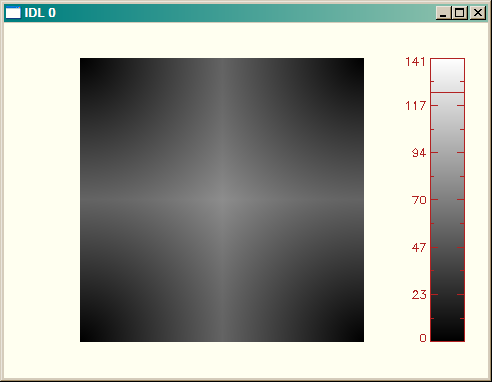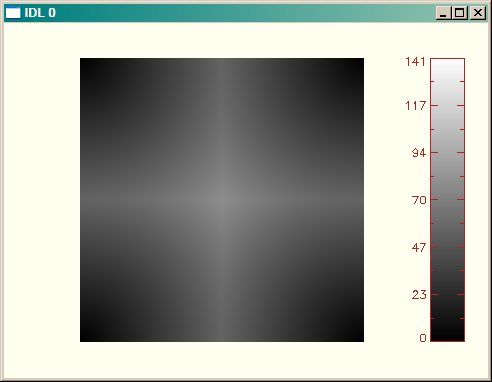# Extra Line in Color Bar

QUESTION: I am using the cgColorbar program from your web page to put a color bar on my display graphic. But there is always an extra line (and always the same color as the color bar annotation) in the color bar. Where does this come from and why is it there? Here is the code I am using.

```   Erase, Color=cgColor('ivory')
image = Dist(200)
s = Size(image, /Dimensions)
pos = [0.1, 0.1, 0.8, 0.9]
cgImage, BytScl(image), Position=pos, /Keep_Aspect
cgColorbar, Range=[Min(image), Max(image)], Color=cgColor('firebrick'), /Vertical
```

You can see the line in the color table in the figure below at value 224.An extraneous line is shown in the color bar.ANSWER: This problem is caused by the color model you are using (indexed color, or color decomposition turned off) in conjuction with the way cgColor and, to a lesser extent, cgColorbar work together. Notice that the problem will go away, at least on your display, by turning color decomposition on:

```   Device, Decomposed=1
Erase, Color=cgColor('ivory')
image = Dist(200)
s = Size(image, /Dimensions)
pos = [0.1, 0.1, 0.8, 0.9]
cgImage, BytScl(image), Position=pos, /Keep_Aspect
cgColorbar, Range=[Min(image), Max(image)], Color=cgColor('firebrick'), /Vertical
```

Of course, this won't help you much if you are using a device, such as the PostScript device, which can only use an indexed color model. So it will help to understand the problem at a deeper level.

Suppose you are using an indexed color model. Then cgColor has to turn the color "firebrick" into a color index number and load that particular color at that index in the color table. The index it chooses, given the way you are calling cgColor, is index number 224. Unfortunately, the call to cgColor must be resolved, and the drawing color loaded into the current color table, before IDL can execute the cgColorbar program, which simply uses the colors currently loaded in the color table. Thus, by the time cgColorbar is run, the wrong color is in the color table.

Note that with color decomposition on, cgColor doesn't need to load a color in the color table, it simply returns a 24-bit value that encodes the color and can be used directly. This is why the solution above works. The color table is not changed by the cgColor program and is the same as it was for the cgImage call prior to the cgColorbar call.

This suggests another way to solve this problem, if (and only if) the annotation color for the color bar is in the current color table. It is not, in this case, but suppose you wanted to draw the annotation in black. With color table 0, the black color is color index number 0, so the problem could be "solved" by using color index 0 as the annotation color. This is done by using the second positional parameter to cgColor, which is the index number where the specified color is to be loaded.

```   Device, Decomposed=0
Erase, Color=cgColor('ivory')
image = Dist(200)
s = Size(image, /Dimensions)
pos = [0.1, 0.1, 0.8, 0.9]
cgImage, BytScl(image), Position=pos, /Keep_Aspect
cgColorbar, Range=[Min(image), Max(image)], Color=cgColor('black', 0), /Vertical
```

But, of course, here we want the annotation color to be different from the colors used for the image. When this is the case, it is customary to divide the color table up into "image colors" and "drawing colors" (sometimes called "annotation colors"). We could, for example, use 255 colors for the image and one for the annotation color. The code would look like this.

```   Device, Decomposed=0
Erase, Color=cgColor('ivory')
image = Dist(200)
s = Size(image, /Dimensions)
pos = [0.1, 0.1, 0.8, 0.9]
cgImage, BytScl(image, Top=254), Position=pos, /Keep_Aspect
cgColorbar, Range=[Min(image), Max(image)], NColors=255, \$
Color=cgColor('firebrick', 255), /Vertical
```

You can see the results in the figure below. Note that this code will work correctly no matter what color model you choose to use. Thus, this is the correct way to write device-independent color code when you are specifying the annotation color with cgColor.Now there is no extraneous line shown in the color bar.# cgColorbar Update

The cgColorbar program has been re-written with an AnnotateColor keyword to help solve this problem. Thus, one other way to fix this problem is to use code like this.

```   Erase, Color=cgColor('ivory')